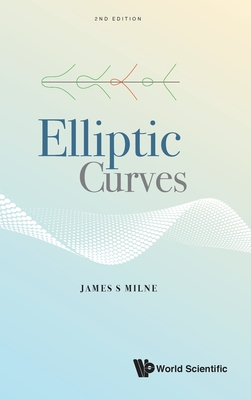### Elliptic Curves,2/e 椭圆曲线（第二版）代数几何学

1015.00

2020年08月21日

ＩＳＢＮ
9789811221835

320 pp

2nd ed.

• 图书详情
• 目次
• 买家须知
• 书评（0）
• 权威书评（0）

This book uses the beautiful theory of elliptic curves to introduce the reader to some of the deeper aspects of number theory. It assumes only a knowledge of the basic algebra, complex analysis, and topology usually taught in first-year graduate courses. An elliptic curve is a plane curve defined by a cubic polynomial. Although the problem of finding the rational points on an elliptic curve has fascinated mathematicians since ancient times, it was not until 1922 that Mordell proved that the points form a finitely generated group. There is still no proven algorithm for finding the rank of the group, but in one of the earliest important applications of computers to mathematics, Birch and Swinnerton-Dyer discovered a relation between the rank and the numbers of points on the curve computed modulo a prime. Chapter IV of the book proves Mordell’s theorem and explains the conjecture of Birch and Swinnerton-Dyer. Every elliptic curve over the rational numbers has an L-series attached to it.Hasse conjectured that this L-series satisfies a functional equation, and in 1955 Taniyama suggested that Hasse’s conjecture could be proved by showing that the L-series arises from a modular form. This was shown to be correct by Wiles (and others) in the 1990s, and, as a consequence, one obtains a proof of Fermat’s Last Theorem. Chapter V of the book is devoted to explaining this work. The first three chapters develop the basic theory of elliptic curves. For this edition, the text has been completely revised and updated. Key Features: o Assumes only the knowledge typically acquired by first-year graduate students o Special care has been taken to place the theory of elliptic curves, both in its historical context and in the context of current research

• 上一个
• 下一个